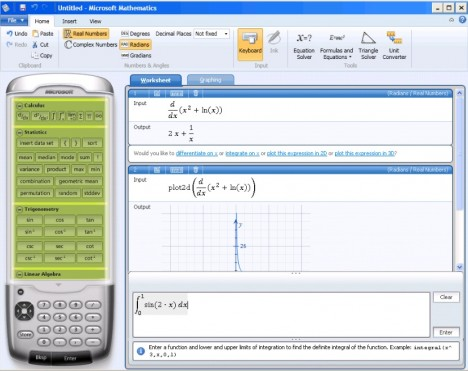This article should be useful for the folks who are struggling to complete the mathematics school work or assignments. In contrast to Microsoft Mathematics add-in, Microsoft Mathematics 4.0 is a free standalone software which focuses in solving mathematical problems ranging from basic mathematics to complicated precalculus. Microsoft Mathematics is an award-winning education tool consisting various powerful mathematical modules to aid in tackling the pre-algebra, algebra, trigonometry, calculus, physics and chemistry calculations.Instead of just deriving mathematical solutions in a blackbox manner, the full-featured graphing calculator, unit converter and step-by-step assistance are the interactive features in Microsoft Mathematics to assist students in learning  the fundamentals of resolving mathematical problems. Explore the  features available in Microsoft Mathematics 4.0:

• Step-by-Step Equation Solver – An interactive assistance to aid the process of resolving complicated problems
• Graphing Calculator – Enable the users to visualize large data-sets in 2D and enhanced 3D color graphs
• Formulas and Equations Library – Eliminate unnecessary memorizations or book flipping as more than 100 commonly used equations and formulae are handily available
• Triangle Solver – A special graphing tool to aid in resolving trigonometry-based problems.
• Unit Conversion tool – Easy conversion involving length, area, volume, weight, temperature, pressure, energy, power, velocity, and time.

In case you are not aware, the previous versions of Microsoft Mathematics which was known as Microsoft Math was a standalone purchasable product which require product activation.